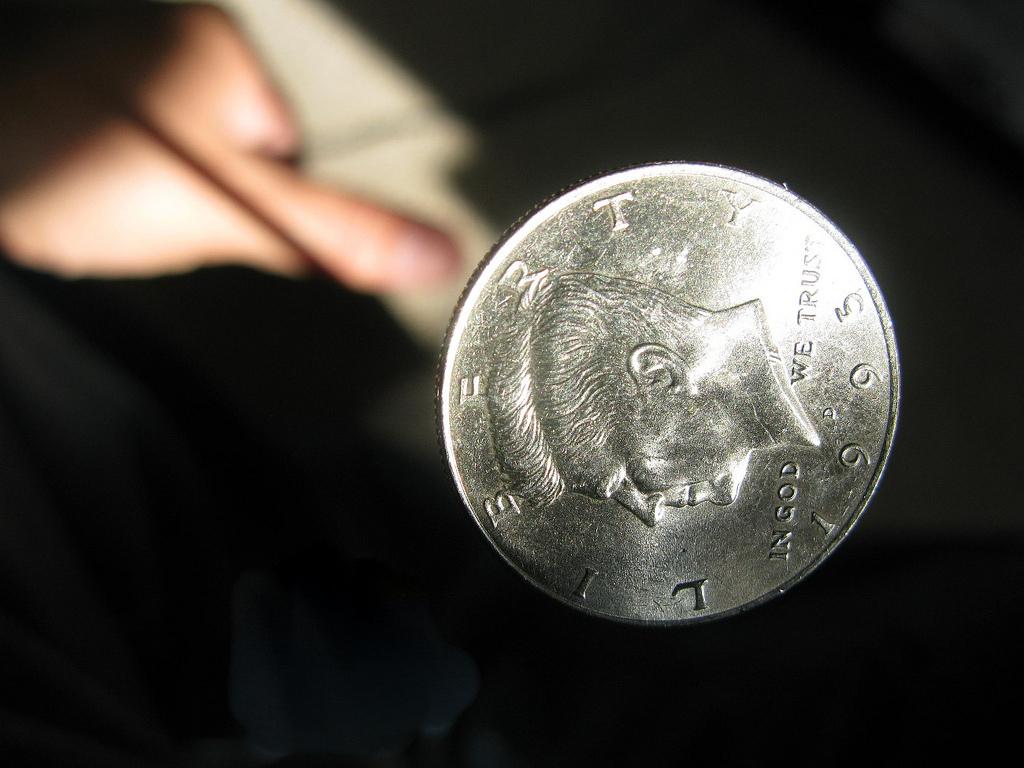## ________________

When the team members can’t think of anything else to do, there are always coin-flipping games.

## ________________Hadley and Taylor play a game in which they flip coins in unison until each of them has flipped tails at least once. (They both continue flipping each time until this happens, so that they always make the same number of flips.) At that point, the winner is the person with the greater ratio of heads flipped to tails flipped.

What is the probability that Hadley is the winner?

### Balanced Flip

If you flip some number of coins simultaneously, call it a balanced flip if exactly half of the coins come up heads.

What number of coins less than 101 maximizes the chance of a balanced flip?

## Solutions to week 100

Average Triangle. Let’s call the initial three labels a, b, and c, with a the smallest and c the largest. (It doesn’t matter if some are equal, so long as none are smaller than a or larger than b.) Then the labels of the second triangle are, in order, (a+b)/2, (a+c)/2, and (b+c)/2. Therefore, the difference of the smallest and largest is now (b+c)/2 – (a+b)/2 = (c+bba)/2 = (ca)/2, or exactly half of what it was on the initial triangle. Similarly, the difference between the smallest and largest label is cut in half on every successive triangle.

But whatever the initial labels were, since they were between 0 and 2017, the initial difference between the largest and smallest label must be less than 2017. Since two raised to the eleventh power is 2048, that means that after that difference has been cut in half eleven times, the difference between the largest and smallest label on the twelfth triangle must be less than one. So now we know the largest label is an integer which differs from 100 by an amount less than one. Hence, the largest label must be 100 as well.

Monotone 5. The key fact to realize here is that given any collection of five distinct nonzero digits, there is exactly one way to make a monotonous number from them (by putting them in ascending order). Hence, we can figure out how many five-digit monotonous numbers there are that start with 1: exactly as many as there are ways to choose the other four digits. But there are eight possible digits to use (2, 3, 4, 5, 6, 7, 8, 9), so there must be eight choose four = 8×7×6×5/4×3×2 = 70 five-digit monotonous numbers that start with 1. So the 71st starts with 2. How many of those are there? Clearly seven choose four = 7×6×5×4/4×3×2 = 35. Hence the largest monotonous five-digit number starting with 2 is the 105th smallest out of all monotonous five-digit numbers. That would be 26,789, and counting back from there, the 104th smallest is 25,789, the 103rd smallest is 25,689, the 102nd smallest is 25,679, the 101st smallest is 25,678, and the 100th smallest is 24,789.

## Recent Weeks

Links to all of the puzzles and solutions are on the Complete Varsity Math page.

Come back next week for answers and more puzzles.